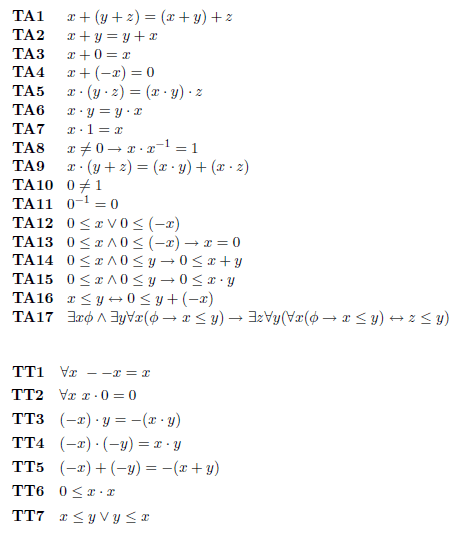# Prove: (x ≤ y) → (x+z ≤ y+z)

[LOGIC] Prove: (x ≤ y) → (x+z ≤ y+z)

I need to prove if x≤y then x+z ≤ y+z (for all x, y and z)

Using these axioms (The first 17 are Tarski Arithmetic, and the following 7 are previously proved results)All I can think of so far is using Axiom TA16, but then what?

Thanks

Last edited:

HallsofIvy
Homework Helper
Yes, that is one way to do it. Note that what you want to end with, $x+z\le y+ z$ is, again by TA16, equivalent to $0\le (y+ z)- (x+ z)$. Do you see how to get to that?

Thanks!

Got that one now

For another question on this example sheet I've almost done it apart from the last step where I have to show

y + (-x) = 0 → y = x

It seems so simple yet I can't think how to show that, any ideas?

Basically I think I need to prove the lemma

x + z = y + z -> x = y